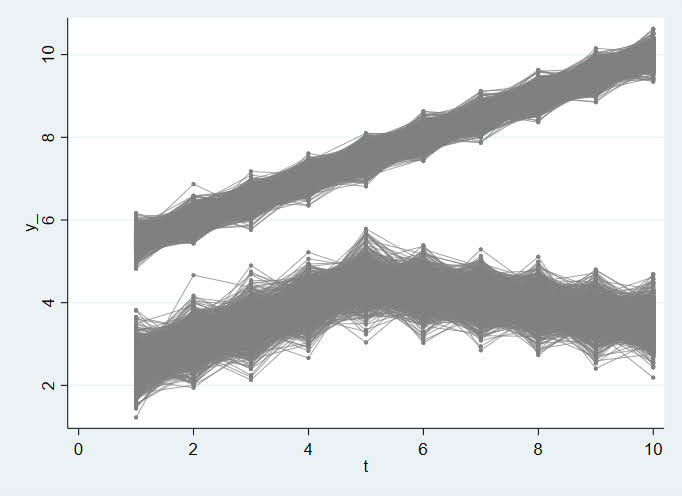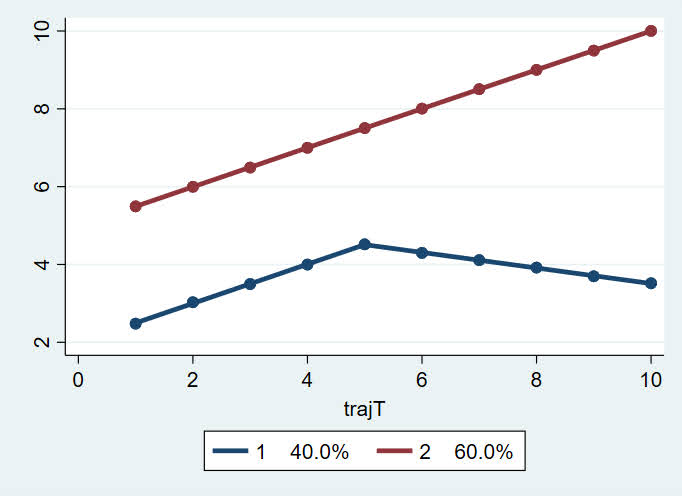# Splines in Stata traj models

On my prior trajectories using Stata post, Nandita Krishnan asks if we can estimate trajectory groups using linear splines (instead of polynomials). I actually prefer restricted cubic splines over polynomials, so it wouldn’t bother me if people did these as a default replacement for the polynomial functions. Also see this paper by Francis et al. using b-splines.

In the Stata traj library, you can just use time varying covariates to swap out the higher order polynomials with the spline effects (whether linear, non-linear, cyclic, etc.) you want. Below is a simple example using linear splines.

# Example Linear Spline

First as a note, I am not the author of the traj library, several people have commented about issues installing on my prior blog post. Please email questions to Bobby Jones and/or Dan Nagin. The only update to note, when I updated Stata to V17 and re-installed traj, I needed to specify a “https” site instead of a “http” one:

``````net from https://www.andrew.cmu.edu/user/bjones/traj
net install traj, force``````

That is about the only potential hint I can give if you are having troubles installing the add-on module. (You could download all the files yourself and install locally I imagine as well.)

Now here I make a simple simulated dataset, with a linear bend in one group and the other a simple line (with some error for both):

``````clear
set more off
set seed 10
set obs 2000
generate caseid = _n
generate group = ceil(caseid/1200) - 1

forval i = 1/10 {
generate y_`i' = 5 + 0.5*`i' + rnormal(0,0.2) if group==0
replace y_`i' = 2 + 0.5*`i' + rnormal(0,0.4) if group==1 & `i'<6
replace y_`i' = 4.5 + -0.2*(`i'-5) + rnormal(0,0.4) if group==1 & `i'>=6
}

* Check out the trajectories
preserve
reshape long y_ , i(caseid) j(t)
graph twoway scatter y t, c(L) msize(tiny) mcolor(gray) lwidth(vthin) lcolor(gray)``````So now we are going to make our spline variables. It is actually easier in long format than wide. Here I do a linear spline, although in Stata you can do restricted cubic splines as well (just google “mkspline Stata” to get the nice PDF docs). So I make the two spline variables, and then just reshape back into wide format:

``````mkspline s1_ 5 s2_=t, marginal
reshape wide y_ s1_ s2_, i(caseid) j(t)``````

And now we can estimate our trajectory model, including our additional `s2_*` linear spline as a time-varying covariate:

``traj, var(y_*) indep(s1_*) model(cnorm) min(0) max(20) order(1 1) sigmabygroup tcov(s2_*)``

And here is the output:

``````==== traj stata plugin ====  Jones BL  Nagin DS,  build: Mar 17 2021

2000 observations used in the trajectory model.

Maximum Likelihood Estimates
Model: Censored Normal (cnorm)

Standard       T for H0:
Group   Parameter        Estimate        Error     Parameter=0   Prob > |T|

1       Intercept         1.99290      0.01432         139.183       0.0000
Linear            0.50328      0.00395         127.479       0.0000
s2_1             -0.70311      0.00629        -111.781       0.0000

2       Intercept         4.98822      0.00581         859.016       0.0000
Linear            0.50310      0.00160         314.224       0.0000
s2_1             -0.00382      0.00255          -1.498       0.1341

1       Sigma             0.40372      0.00319         126.466       0.0000
2       Sigma             0.20053      0.00129         154.888       0.0000

Group membership
1       (%)              40.00001      1.09566          36.508       0.0000
2       (%)              59.99999      1.09566          54.761       0.0000

BIC= -3231.50 (N=20000)  BIC= -3221.14 (N=2000)  AIC= -3195.94  ll=  -3186.94

Entropy = unknown function /log()``````

Not sure about the unknown function log error message. But otherwise is what we expected. Surprisingly to me `trajplot` works out of the box:

``trajplot``I figured I would need to write matrix code to get this plot, but no issues! If you are wondering why is the estimated spline effect here ~-0.7 instead of -0.2 (how I simulated the data), it is because these are cumulative effects, not independent ones. To get the -0.2, you can do post-hoc Wald tests:

``````matrix list e(b)
lincom _b[s2_1G1] + _b[linear1]``````

Which gives as output:

``````. matrix list e(b)

e(b)[1,9]
interc1     linear1      s2_1G1     interc2     linear2      s2_1G2      sigma1      sigma2      theta2
y1   1.9928963   .50327955  -.70311464   4.9882231   .50310322  -.00382206   .40372185   .20052795   .40546454

.
. lincom _b[s2_1G1] + _b[linear1]

( 1)  linear1 + s2_1G1 = 0

------------------------------------------------------------------------------
| Coefficient  Std. err.      z    P>|z|     [95% conf. interval]
-------------+----------------------------------------------------------------
(1) |  -.1998351    .003097   -64.52   0.000    -.2059051    -.193765
------------------------------------------------------------------------------``````

While this is for a linear spline, more complicated cubic splines (or whatever you want, you could do cyclic splines if you have repeating data for example) will just result in more variables going into the `tcov()` parameters.

Note a major limitation of this, you need to specify the spline knot locations upfront. The method does not do a search for the knots – here I needed to tell `mkspline` to set a knot location at t=5. I think this is an OK limitation, as specifying knots at regular locations along the domain of the data can approximate many non-linear functions, and it is not much different than assuming a specific number of polynomials (but will tend to behave better in the tails).

Alternate models search for the changepoints themselves, but that would be tough to extend to mixture models (quite a few unknown parameters), better to either have flexible fixed splines, or just go for a continuous multi-level model formulation instead of discrete mixture classes.

1.#### Pauline BD

/  January 19, 2023

Dr. Wheeler,

At the beginning of the blog, you wrote:

I actually prefer restricted cubic splines over polynomials, so it wouldn’t bother me if people did these as a default replacement for the polynomial functions. Also see this paper by Francis et al. using b-splines.

What the difference between restricted cubic splines and b-splines? Can you recommend any references introducing b-splines.

I am new to spline modelling.

Thanks!

Pauline

2.